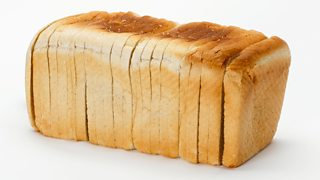Understanding ratio

A ratio shows the relationship between two quantities.

It is written in the form a:b which is read as ‘a to b’.

Example

Jon has a pack of sweets. He shares them with a friend in the ratio 3:2. This means that for every three sweets John eats, he gives his friend two.

If Jon eats 15 sweets (5 × 3), his friend will receive 10 sweets (5 × 2).

In the example, this means that Jon has 5 lots of 3 sweets so his friend will have 5 lots of 2 sweets.

QuestionCREDIT: SSPL/Getty Images

Bread is made using five parts of flour to three parts liquid.

a) Write down the ratio of liquid to flour.

b) If the recipe calls for 200 g of flour, how much liquid will be needed?

a) 3:5

As the question asks for the ratio of liquid to flour, you must write the number of parts of liquid first, followed by flour.

b) Five parts = 200

One part = 200 ÷ 5 = 40

Three parts = 3 × 40 = 120

120 ml of liquid will be needed.

Question

Cookies are made using the ratio 3:2:1 of flour, fat and sugar.

If a recipe calls for 150 g of flour, how much fat and sugar are needed?

• Three parts = 150 g
• Divide 150 by three to find what one part is worth
• One part = 50 g
• Fat = Two parts = 2 × 50 = 100 g
• Sugar = One part = 50 g